Enhance Your Plotly Express Scatter Plot With Marginal Plots

Display Extra Information on your Plotly Express Scatter Plots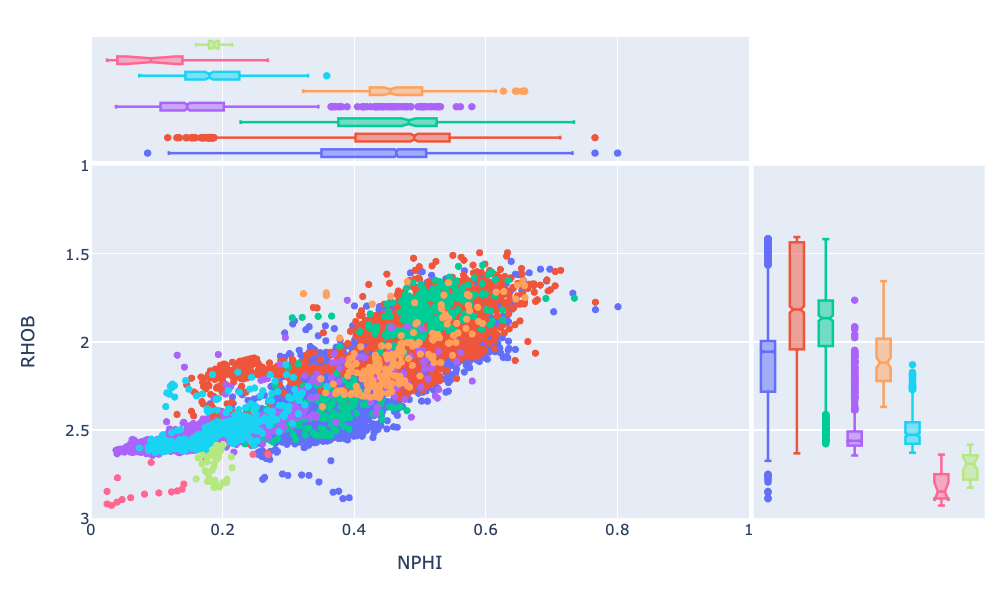Plotly Express Scatter Plot of well log data illustrating marginal plots on both axes. Image by the author.

Scatter plots are a commonly used data visualisation tool within data science. They allow us to plot two numerical variables, as points, on a two dimensional graph. From these plots, we can understand if there is a relationship between the two variables, and what the strength of that relationship is.

Using Plotly Express to Create Interactive Scatter Plots

An Example of Creating Interactive Scatter Plots Using Well Log Data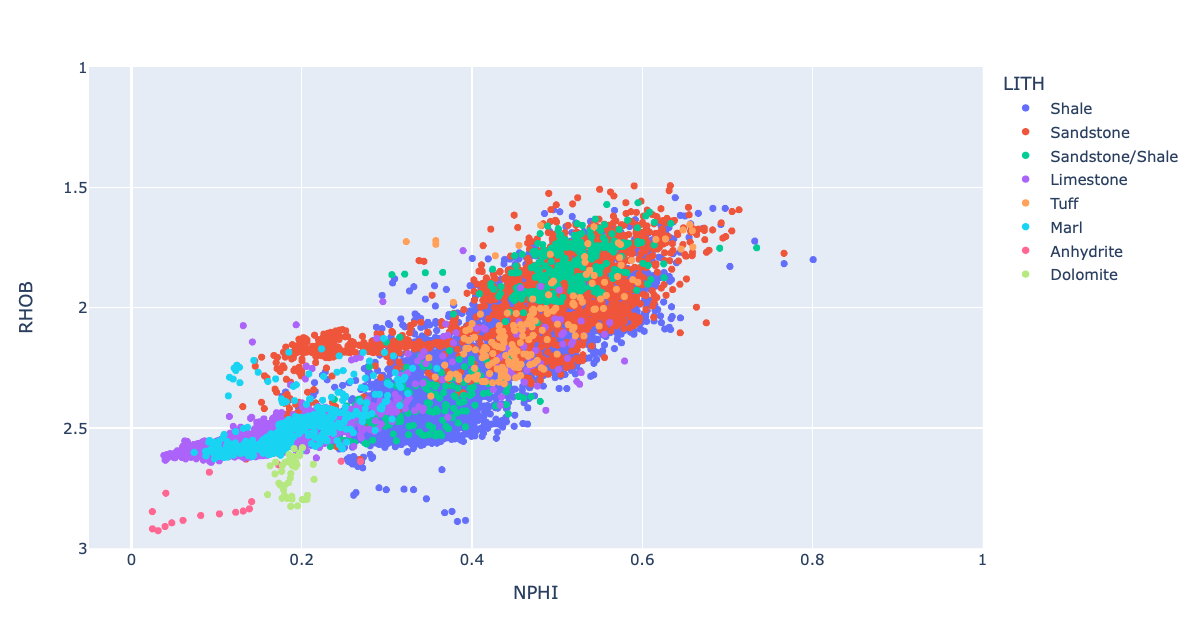Plotly Express scatter plot of well log data. Image by Author.

Scatter plots allow us to plot two variables from a dataset and compare them. From these plots, we can understand if there is a relationship between the two variables, and what the strength of that relationship is.

Within petrophysics scatter plots, are commonly known as crossplots. They are routinely used…

Visualizing Well Paths With The Welly Python Library

Creating 3D Line Plots Using Matplotlib to Visualize Well Trajectories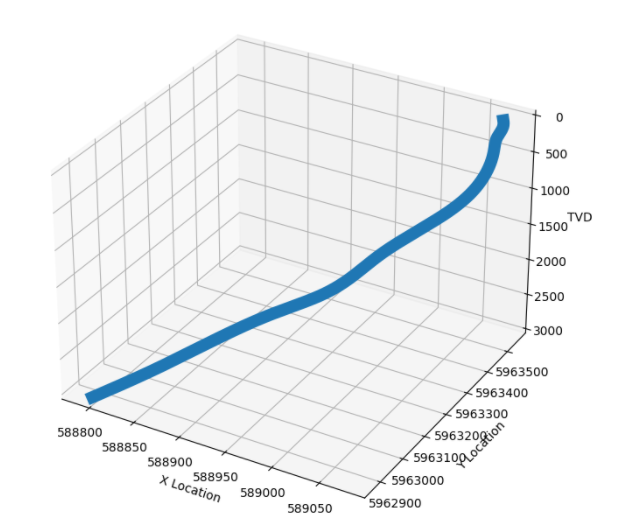3D Line Plot showing the position of a well path generated from the Welly Python Library. Image by the author.

There are multiple depth references used within well logging to denote a position along the wellbore. These include measured depth (MD), true vertical depth (TVD), true vertical depth subsea (TVDSS) etc. All of which are critical measurements for the successful development and completion of a well.

Well Log Data Outlier Detection With Machine Learning and Python

Identification of outliers is an essential step in the machine learning workflow

Outliers are anomalous points within a dataset. They are points that don’t fit within the normal or expected statistical distribution of the dataset and can occur for a variety of reasons such as sensor and measurement errors, poor data sampling techniques, and unexpected events.

Within well log measurements and petrophysics…

Identification and Handling of Missing Well Log Data Prior to Petrophysical Machine Learning

Part 2 in a series going from Exploratory Data Analysis to Machine Learning with Well Log Data

Machine learning and Artificial Intelligence are becoming popular within the geoscience and petrophysics domains. Especially over the past decade. Machine learning is a subdivision of Artificial Intelligence and is the process by which computers can learn and make predictions from data without being explicitly programmed to do so. …

Exploring Well Log Data Using Pandas, Matplotlib, and Seaborn

An example of exploring petrophysical and well log measurements using a number of plots from Seaborn and Matplotlib

Machine learning and Artificial Intelligence are becoming popular within the geoscience and petrophysics domains. Especially over the past decade. Machine learning is a subdivision of Artificial Intelligence and is the process by which computers can learn and make predictions from data without being explicitly programmed to do so. …

How to Rename Columns in Pandas — A Quick Guide

A short guide on multiple options for renaming columns in a pandas dataframe

Ensuring that dataframe columns are appropriately named is essential to understand what data is contained within, especially when we pass our data on to others. In this short article, we will cover a number of ways to rename columns within a pandas dataframe.

But first, what is Pandas? Pandas is…

Creating Boxplots Using Matplotlib in Python

Understand your data distribution and identify outliers in petrophysics and well log data using boxplots

Boxplots are a great tool for data visualisation, they can be used to understand the distribution of your data, whether it is skewed or not, and whether any outliers are present. …

Using Line Plots from Matplotlib to Create Simple Log Plots of Well Log Data

Visualising well log data versus depth using the matplotlib library from Python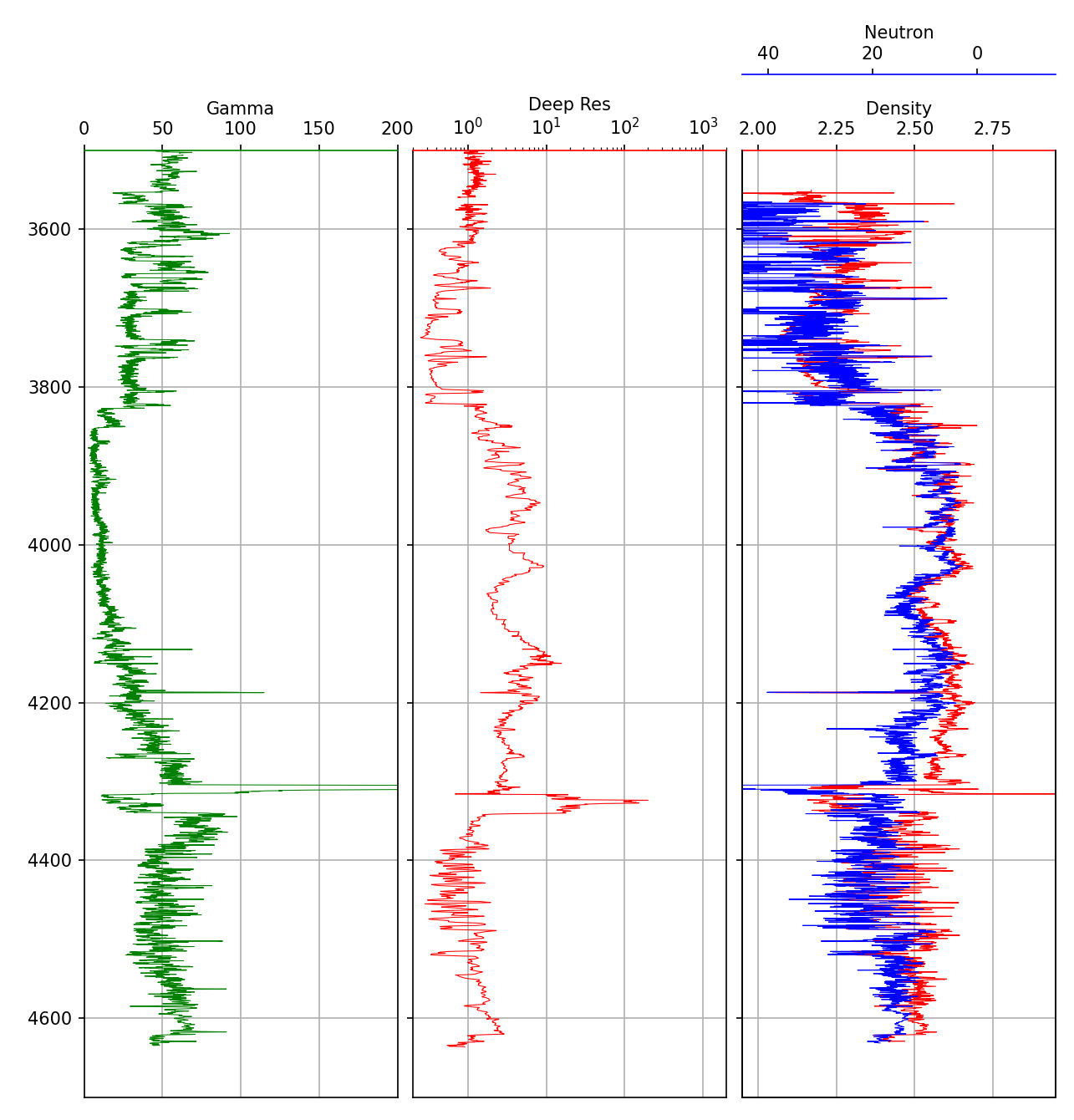Well log plot created using the matplotlib Python library. Image by author.

Introduction

Well log plots are a common visualization tool within geoscience and petrophysics. They allow easy visualization of data (for example, Gamma Ray, Neutron Porosity, Bulk Density, etc) that have been acquired along the length (depth) of a wellbore. …

Creating Scatter Plots (Crossplots) of Well Log Data using matplotlib in Python

Use scatter plots to visualise the relationship between variables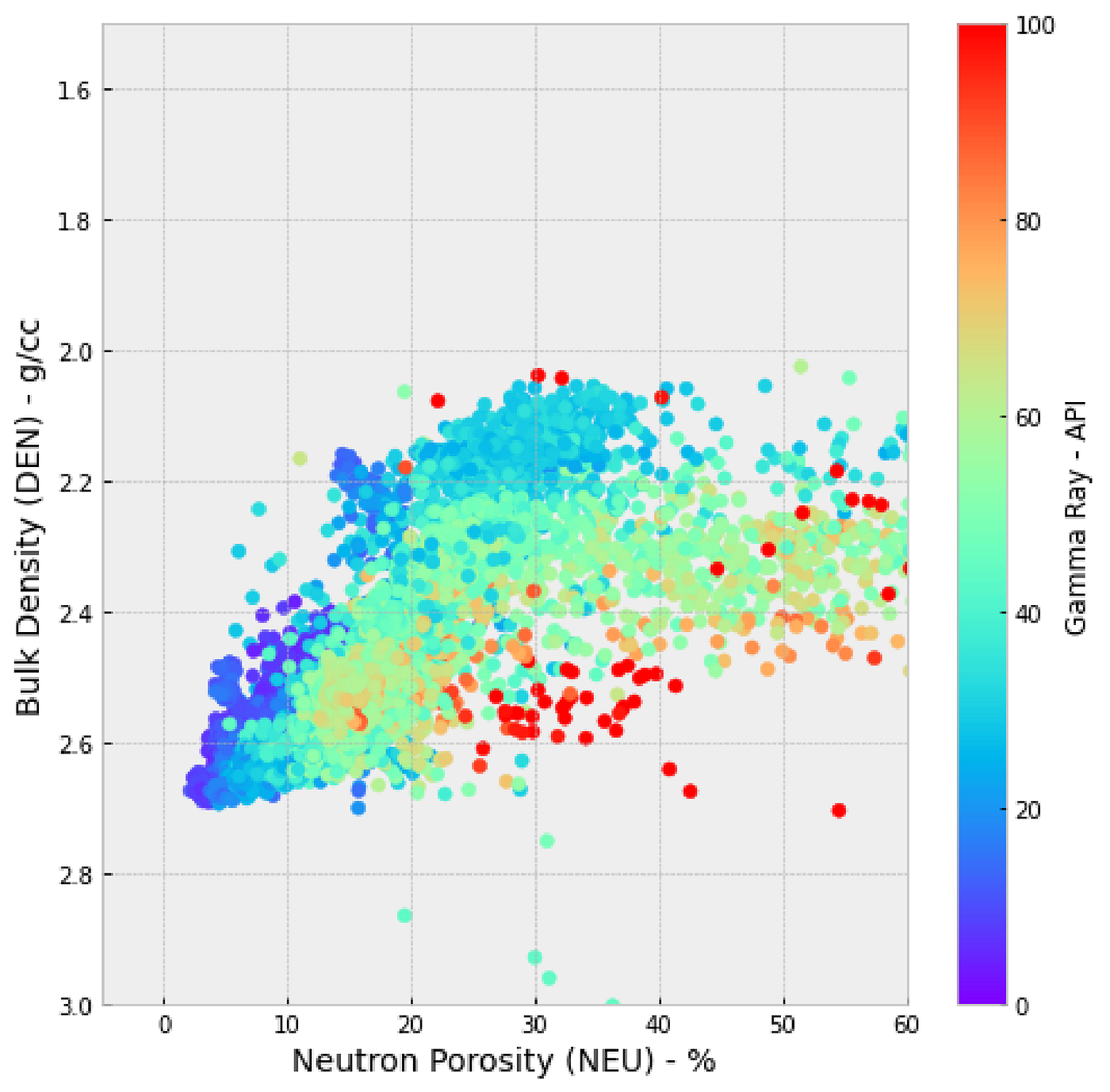Neutron density scatter plot / crossplot created with matplotlib in python. Image by the author.

Introduction

Scatter plots are a commonly used data visualisation tool. They allow us to identify and determine if there is a relationship (correlation) between two variables and the strength of that relationship.

Within petrophysics scatter plots, are commonly known as crossplots. …Andy McDonald

Petrophysicist, Geoscientist and data Scientist with a passion for data analytics, machine learning, and artificial intelligence.